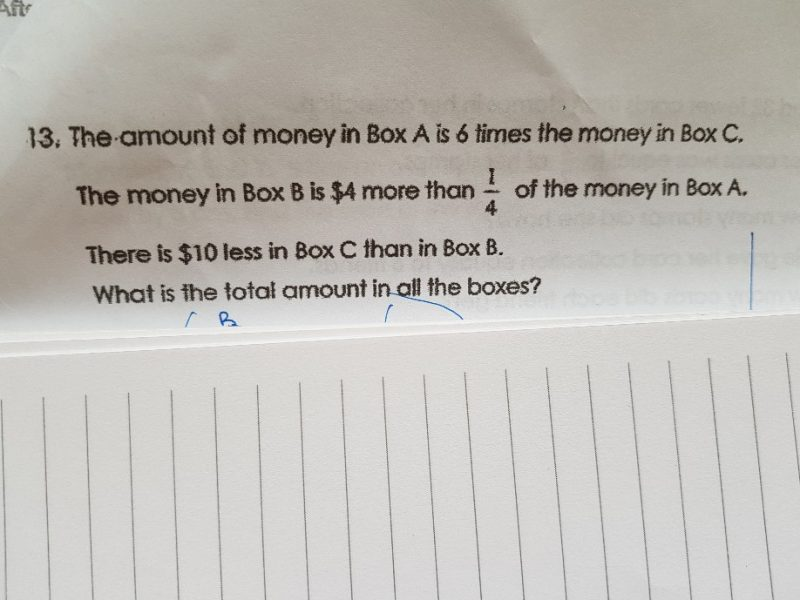# QuestionLet Box A contain 12 units.

Then Box C contains 2 units.

Box B contains 3 units + 4.

Box B contains \$10 more than Box C, so 3u + 4 = 2u + 10

1u = 6

Total in the 3 boxes = 12u + 3u + 4 + 2u = 17u + 4 = 17  × 6 + 4 = 102 + 4 = 106

The total amount is \$106.

Hi thw,

At first, how do you know box A contain 12 units?

Thanks.

Box A has 6 times as much as C. But 6 is not divisible by 4 so we use 12 units.

Then C is 1/6 of 12 units = 2 units.